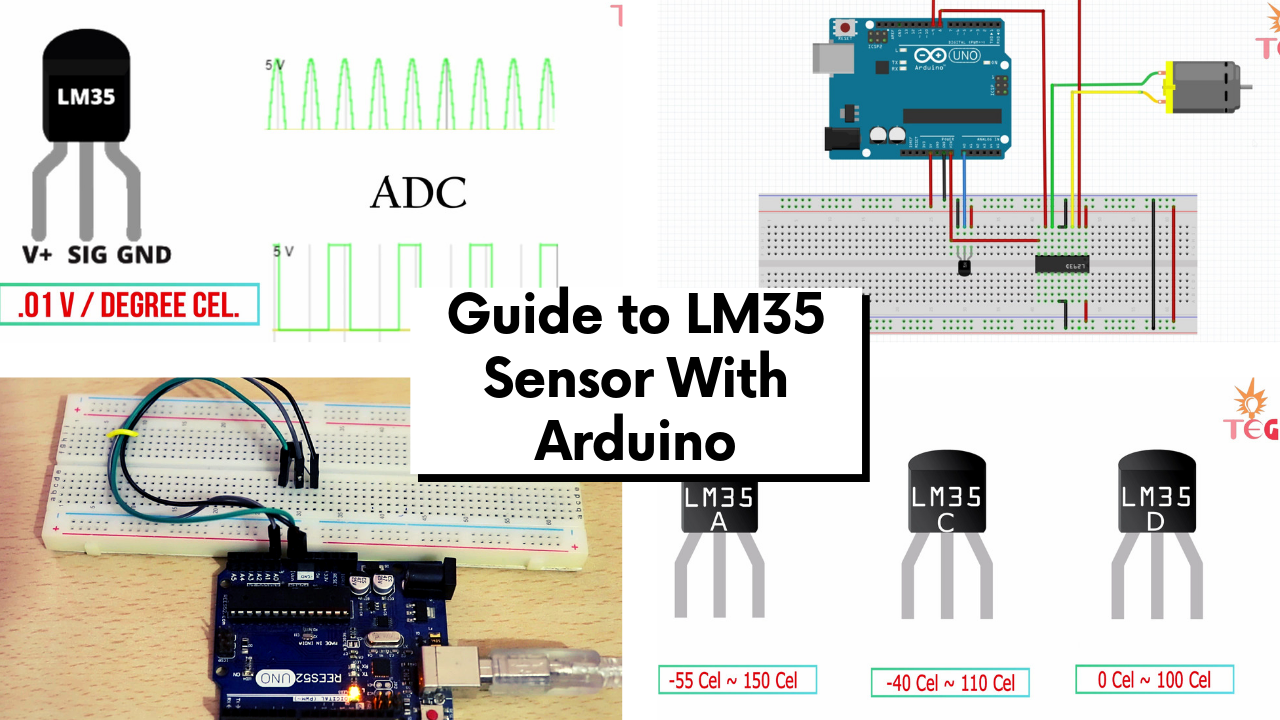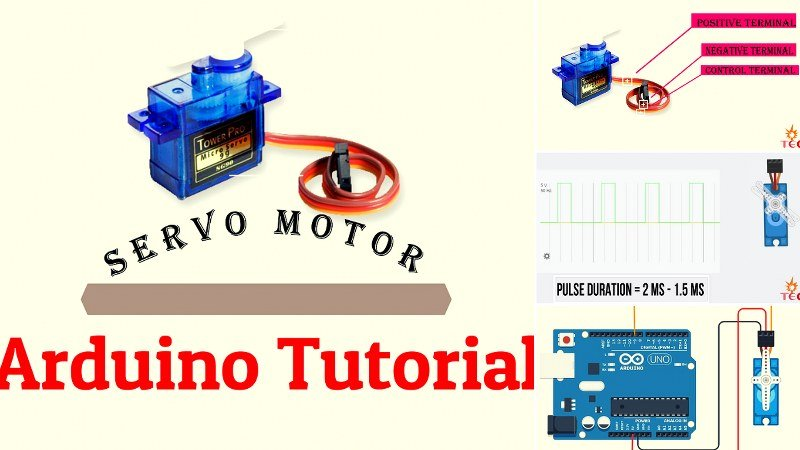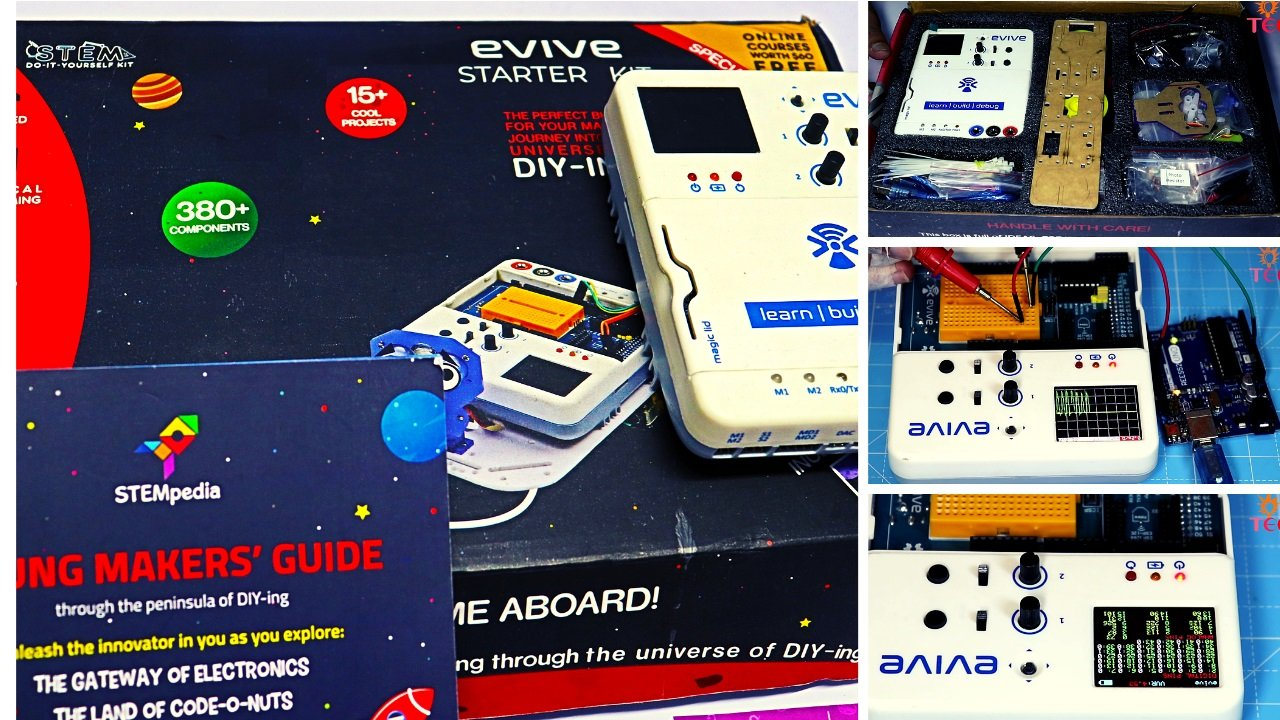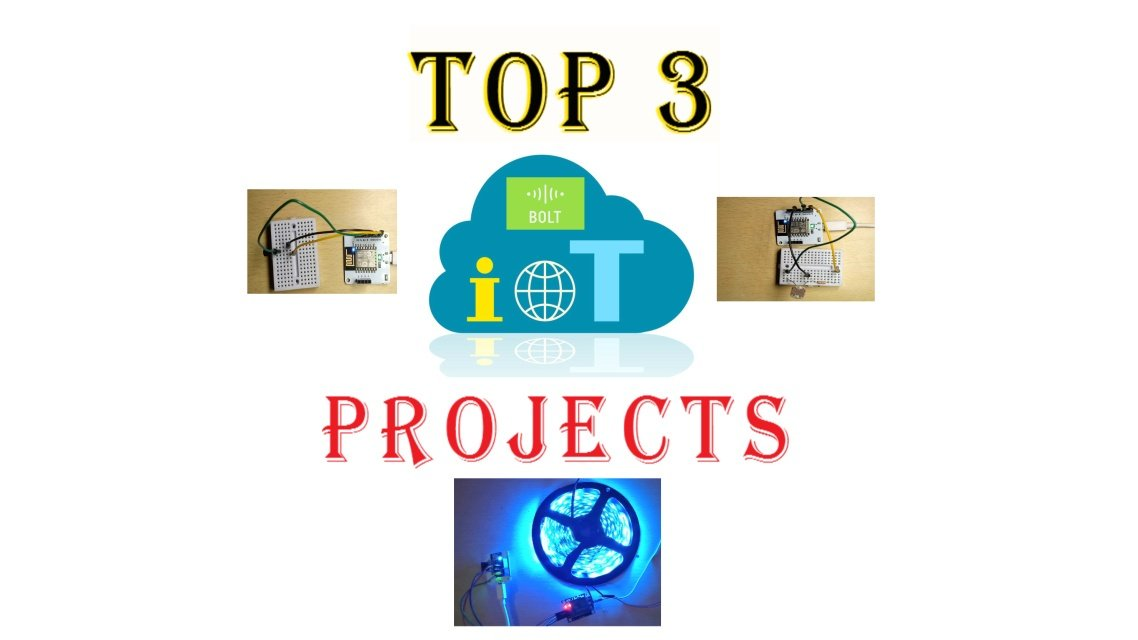# Beginners Guide to LM35 with Arduino and workingThis is a detailed tutorial on LM35 temperature sensor for beginners. You will learn the basics of LM35, it’s working and how to interface LM35 with Arduino.

1) INTRODUCTION TO LM35 TEMPERATURE SENSOR

LM35 is an analog, linear temperature sensor. Analog means the output voltage of LM35 is analog in nature and linear means this output voltage varies linearly with the change in temperature.

NOTE: You can also watch this “LM35 Beginners guide” video for better understanding or read the written tutorial below:

LM35 is a 3 terminal device and here is the pinout of LM35:

2) PINOUT OF LM35

1.  +Vcc and ground provides power to the sensor. You can supply any voltage between 4v to 20v.

2.  Vout OR signal pin gives the output voltage corresponding to temperature. And the scale factor is .01v rise per degree Celsius.

Since LM35 is an analog device and gives analog output, the output pin of LM35 should be connected to the ADC aka analog to digital converter first to convert the output analog signal to Digital. Now the good thing is Arduino has its own inbuilt ADC. So we need to just connect the Analog pin of Arduino to Vout pin of LM35.

Another important factor to consider when using LM35 in your project is its low self-heating due to its drawing current which is only 60 uA.

3) Types of LM35 temperature sensors available:

There are three different variants of LM35 available in a transistor package. LM35A, LM35C, and LM35D. The only difference being in the range of temperature measurements.

1) LM35A can measure temperature between -55 to 150 degree Celsius.

2) LM35C between -40 to 110 and

3) LM35D between 0 to 100 degree Celsius. In this tutorial, I am using the LM35DZ variant.

4) Functional Block Diagram of LM35:

This is the functional block diagram of a typical LM35 which you can find in its datasheet. And the three pins are shown below:

What this circuit does is:

1) It gives a temperature-dependent voltage output at N terminal of the diode buffered by amplifier A2 to give output to Vout pin.

2) These two transistors are used to create a bandgap voltage reference i.e, a constant fixed voltage reference regardless of temperature and power supply variations.

3) And this is the reason you can power LM35 using any voltage between 4 to 20v.

Now let’s see how to interface temperature sensor with Arduino to print the surrounding temperature on Serial monitor of Arduino IDE

5) Circuit Connections of Temperature measurement using LM35 with Arduino:

1.  +Vcc of LM35 goes to 5 v pin of Arduino

2. GND of LM35 goes to GND pin of Arduino

3. And Vout goes to the Analog pin A0.

I have already mentioned that the analog output of the temperature sensor should be converted to digital-first and the scale factor is .01v rise per degree Celsius. So we are going to use an analog pin of Arduino to convert this analog output voltage values to digital values and then by using a formula in the program, convert these digital values to the corresponding temperature.

6) Analog output to temperature in Degree Celsius conversion formula:

A. Suppose we are getting a value of 65 on Serial monitor from the Vout pin of LM35.

B. We know 1023 corresponds to 5v in Arduino. So 350 will corresponds to (5/1023)* 65 and this will gives us the output voltage.C. To convert this voltage to temperature in degree Celsius, we are going to use the scale factor of .01V rise per degree Celsius.

D. 1v gives us 100 degrees Celcius so the calculated voltage will give: Calculate voltage multiplied by 100. And this is the surrounding temperature in degree Celsius.

We are going to use this formula in our program. Here is the program you need to write for the same:

7) Program: Arduino LM35 temperature measurement

```float Vout;
float Temp;
void setup()
{ Serial.begin(9600);
}

void loop()
{
Temp= (Vout*500)/1023 ;
Serial.print("Temperature in Degree Celsius = ");
Serial.print(Temp);
Serial.println();
delay(1000);
}```

A. First store the value from analog pin A0 to the Vout variable.

B. Then by using the formula explained earlier convert this value to degree Celsius.

C. And print these temperature values onto the serial monitor by using simple Serial commands.

Connect Arduino burn cable to your laptop, select COM port and upload the code. Now open the serial monitor. The room temperature gets printed onto the serial monitor. Try to bring the hot iron close to the surface of LM35 and observe the change in temperature.

 Read Also: Beginners Guide to Servo Motors before this LM35 sensor Guide.

8) Temperature controlled DC fan state using LM35 Sensor and Arduino :

Here’s a small project you can easily make to control the state of the DC motor according to a predefined temperature limit. What this means is that as soon as the temperature rises to a certain value DC motor will start running. Please keep in mind that we are not controlling the motor speed here. It is just state control.

For this small project apart from Arduino and LM35 temperature sensor, you will also need a motor driver L293D(L298N will also do the job), 6-12v DC motor and external power supply.

Here are the circuit connections for the same:

Now, this circuit has a Motor driver IC to control the motor. So we are going to use the L293D Module or L293D IC. You can also use L298N for the same purpose.

9) Pinout of L293D Motor driver IC:

Here is the pinout of L293N IC having DIP package with 16 pin

1. Enable 1: is the pin which enables OUT1 and OUT2 either ON or OFF i.e in order to use these pin as output enable pin must be set HIGH. Similarly, Enable 2 for      OUT3 and OUT4.

2. IN1:  is the pin connected to the digital output pin of Arduino to control OUT1(Either ON or OFF). Similarly IN2, IN3, and IN4 for OUT2, OUT3, and OUT4         respectively.

3. 0V pin: Pin 4,5,13 and 12 should be connected to the Gnd of Arduino.

4. OUT 1 & OUT 2: is directly connected to the terminals of Motor 1.

5. +V motor: This pin is connected to the external power supply to directly power the motor. In our case, the Vin pin of Arduino since we are going to use external 12 v adapter to power Arduino

6. OUT 3 & OUT 4: is directly connected to the terminals of Motor 2.

7. +V: The 16th pin is connected to 5v pin of Arduino to power the IC.

NOTE: To make things easier I am going to use the L293D Motor driver module instead of IC. But if you are a beginner I will recommend using L293D IC.

10) Program: Temperature controlled DC fan state using LM35:

This is the Program you need to write for the same:

```float Vout;
float Temp;

void setup() {

Serial.begin(9600);
pinMode(8,OUTPUT);
pinMode(9,OUTPUT);

}

void loop() {
Temp= (Vout*500)/1023 ;
if(Temp>=40)
{
digitalWrite(8,HIGH);
digitalWrite(9,LOW );
}

else{ digitalWrite(8,LOW);
digitalWrite(9,LOW );
}
Serial.print("Temperature in Degree Celsius = ");
Serial.print(Temp);
Serial.println();
delay(1000);

}```

1)  First, set digital pin 8 and 9 as OUTPUT pins

2) Store the value from analog pin A0 to the Vout variable. Then by using the formula explained earlier convert this value to degree Celsius.

3) Now we are going to use if command to set the condition to turn the motor on. So if the temperature is >=40 then Digitalwrite 8 pin high and 9 pin Low

4) Else in any other case Digital write both the pins LOW.

What this program does is that, when the temperature is less than 40 degree Celsius, Motor will not run but as soon as the temperature hits 40-degrees Motor starts running.

NOTE:  Upload this code and power the Arduino from external 12 v Adapter.

1)  Open the serial monitor. As you can see temperature right now is around 30 degree Celsius which is less than 40 degree, Motor is not rotating.

2)  Increase the temperature by bringing heated iron close to the surface of LM35 and observe the Motor state:

So the motor works exactly as we want according to the temperature.

In the next part of this tutorial, we will move forward and see how to control the speed of DC motor according to the temperature rise.

 Read Also: Beginners Guide to Servo Motors before this LM35 sensor Guide.

# Arduino Servo Motor Guide in 2019: All you need to Know about ServosHey Guys welcome back to the another arduino tutorial and in this “Arduino Servo Motor Guide”, you are going to learn about servo motors and how to interface them with arduino. So Let’s get started to this “Arduino Servo Motor Guide in 2019”.

# Evive – Best Arduino prototype tool kit for beginnersHey guys welcome back to the another review tutorial and I have got something cool for you today. I have been playing with this toolkit for about a week now and I must say I am impressed. So now lets get on with the unboxing of “Evive – Best Arduino prototype tool kit for beginners”.

# Why there is more static electricity or static shock in winters?Before answering the question: why there is more static electricity in winters? we first must understand, why does this happen and why do we get a static shock in the first place?# Top IoT Projects for beginners in 2019 using Bolt IoT DeviceHey guys in this tutorial we are going to make 3 easy IoT projects for absolute beginners using Bolt IoT device. So if you are looking for a detailed post on top IoT projects for beginners in 2019, then you crashed at the right webpage.

Our first project will be a light monitoring system which can be used for monitoring the amount of light a plant is receiving.